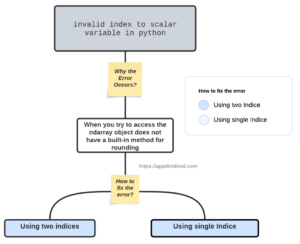# How to Fix IndexError: invalid index to scalar variable in PythonDiagram

Python raises an IndexError: invalid index to scalar variable is a “compile-time error that occurs when you try to access an element of an array or list using incorrect index position or dimension-tier ([][])”.

If you try to access an element from a 2D array using three indices([][][]), you will get the IndexError: invalid index to scalar variable error.

## Reproducing the error

``````import numpy as np

value = np.array([[21, 19], [11, 18], [21, 46]])

print("The value is ", value)``````

Output

``IndexError: invalid index to scalar variable.``

The above code will generate the “IndexError: invalid index to scalar variable” error because the value variable is a 2D array with shape (3,2), and it only has two indices to access the elements, the first for rows and the second for columns.

We are trying to access an additional index by using value where the first index 0 is for rows, the second index 1 is for columns, and the third index 2 is not valid because the value is a 2D array.

## How to Fix IndexError: invalid index to scalar variable

There are the following methods to fix the IndexError in Python.

1. Method 1: Access the element using only two indices, “value ”.
2. Method 2: Access the element using a single index, “value, value, value”.

### Method 1: Using two indices

To access the specific value from a two-dimensional array, use the value syntax.

``````import numpy as np

value = np.array([[21, 19], [11, 18], [21, 46]])

element = value

print("The element is:", element)``````

#### Output

``The element is: 19``

In the above code, the single element is accessed using the indices (0,1), corresponding to the element in the first row and second column of the 2D array.

This code won’t raise the “IndexError: invalid index to the scalar variable” error because the indices (0,1) are valid for the 2D array.

### Method 2: Using single Indice

To access the specific array from a two-dimensional array, use the value syntax.

``````import numpy as np

value = np.array([[21, 19], [11, 18], [21, 46]])

element_first = value
element_second = value
element_third = value

print("The first element is:", element_first)
print("The second element is:", element_second)
print("The third element is:", element_third)``````

Output

``````The first element is: [21 19]
The second element is: [11 18]
The third element is: [21 46]``````

In the above code, the element_first is accessed using the index 0, corresponding to the element in the first row of the 2D array.

The element_second is accessed using index 1, and element_third is accessed using index 2.

The code won’t raise the “IndexError” error because the index 0, 1, and 2 are valid indices for the 2D array.

That’s it.

This site uses Akismet to reduce spam. Learn how your comment data is processed.